# Schrodinger equation

The Schrödinger equation is a linear differential equation used in various fields of physics to describe the time evolution of quantum states. It is the fundamental equation of non-relativistic quantum meachincs. The equation is named after its discoverer, Erwin Schrödinger.

## Mathematical forms

### General time-dependent form

The Schrödinger equation may generally be writtenwhere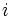is the imaginary unit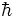is the reduced Planck's constantis the quantum mechanical state or wavefunction (expressed here in Dirac notation)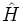is the Hamiltonian operator.

The left side of the equation describes how the wavefunction changes with time; the right side is related to its energy. For the simplest case of a particle of mass m moving in a one-dimensional potential V(x), the Schrödinger equation can be writtenThis can be expanded to give the three dimensional time dependent Schrödinger equation using the Laplacian operator,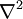: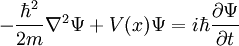In this case, the wavefunction is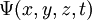.

### Derivation

The quickest and easiest way to derive Schrödinger's equation is to understand the Hamiltonian operator in quantum mechanics. In classical mechanics, the total energy of a system is given bywhere p is the momentum of the particle and V(x) is its potential energy. Applying the quantum mechanical operator for momentum: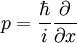and subbing into the classical mechanical form for energy, we get the same Hamiltonian operator in quantum mechanics:from which Schrödinger's equation and the eigenvalue problem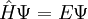can be easily seen.

### Time-independent Schrödinger Equation

The wavefunction is a multi-variable function, that is to say it depends on both time and space. Suppose that we have a function only in terms of space,. We can write the full wavefunction asfor a state of definite energy. This type of state is also known as a "stationary state" as the probability distribution for this type of wavefunction over time is constant (see below). Substituting this wavefunction into the Schrödinger equation:Cancelling the factor ofresults in a differential equation that only depends on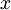. This is the time-independent Schrödinger equation: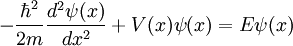is often called the "wavefunction", though strictly speaking it isthat is the wavefunction.

### Solutions to the Schrödinger Equation

Solutions to the Schrödinger equation are known as "wavefunctions". However, for a solution to be physically valid, two conditions must be imposed on these solutions. These are that both the wavefunction and its first derivative must be continuous. Wavefunctions may be real or complex.

Since the Schrödinger equation is linear, ifandare solutions, thenis also a solution.

### Eigenvalue problems

In many instances, steady-state solutions to the equation are of great interest. Physically, these solutions correspond to situations in which the wavefunction has a well-defined energy. The energy is then said to be an eigenvalue for the equation, and the wavefunction corresponding to that energy is called an eigenfunction or eigenstate. In such cases, the Schrödinger equation is time-independent and is often writtenHere, E is energy, H is once again the Hamiltonian operator, andis the energy eigenstate for E.

One example of this type of eigenvalue problem is an electrons bound inside an atom.

### Interpretation of the Wavefunction

The wavefunction is related to the probability of observing a particle at that location in space. More specifically, where the wavefunction has a greater value of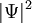at one location, a, compared to another, b, then the particle is more likely to be observed at a than b. Note that the modulus must be taken since the wavefunction may be complex.

#### Normalisation

Wavefunctions are said to be "normalisable" if the following integral exists: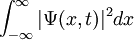If that integral exists, then the wave function may be normalised by introducing a constant,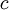, such thatNote thatmay be complex. This will produce and new wavefunction,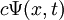, which has the property that: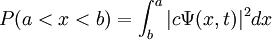This is the probability of observing the particle between points a and b. For some wavefunctions, such as that of a free particle, the integral above does not exist and so they are said to be non-normalisable.

## Examples for the Time-Independent Equation

### Free particle in one dimension

In this case,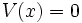, the time-independent Schrödinger equation is:In this case, the solution is sinusoidal for some constants,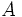,,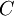, and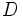:The energy can then be found as: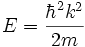Physically, this corresponds to a wave travelling with a momentum given by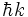, where k can in principle take any value. This wavefunction is not normalisable.

### Particle in a box

Consider a one-dimensional box of width a, where the potential energy is 0 inside the box and infinite outside of it. We can describe this potential by: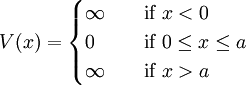This is also known as "the infinite square well". This means thatmust be zero outside the box. One can verify (by substituting into the Schrödinger equation) thatis a solution if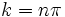where n is any integer. Thus, rather than the continuum of solutions for the free particle, for the particle in a box there is a set of discrete solutions with energies given by### Derivation

The particle cannot exist outside the box where the potential is infinite. Hence the wavefunction,, here must be zero outside the box. Now let us consider inside the box. The Schrödinger equation inside the potential well becomes: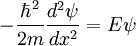Rearranging this equation giveThis has the same form as the basic equation for simple harmonic motion. Hence we can give the solution as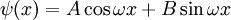whereandare unknown constants and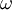is equal to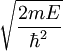. To indicate the value of the constants, we substitute in boundary conditions. An additional constraint is that wavefunctions must be continious (they cannot suddenly change value). HenceFrom this we can see thatmust be zero. Using the continuous constraint again, we see that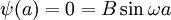The continuous constraint is only satisfied when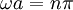whereis an integer. We also note that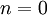is not a solution as the wavefunction would be zero everywhere and so the probability of finding the particle is 0. We also discard solutions for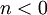since these are not "new" solutions. As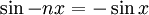, these are just the negative of solutions with positive. By setting, we can find the energyas: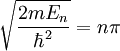And so: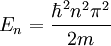You may wonder what happens about the constant. Since the Schrödinger equation is linear,can take any value and the resulting wavefunction would be a solution. Since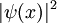represents the probability of finding the particle at positionand across all space, this probability must equal 1, we can solve for. We do this by integratingfromto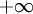. Therefore, the full solution is:whereis as above.

## Relativity

The Schrödinger equation is non-relativistic in nature. It can be re-derived taking special relativity into account. This results in the Klein-Gordon equation.